```
Digital  interview questions Page 1   Page 2

Page 3  Page 4 Page 5
```

48) Draw timing diagrams for following circuit.?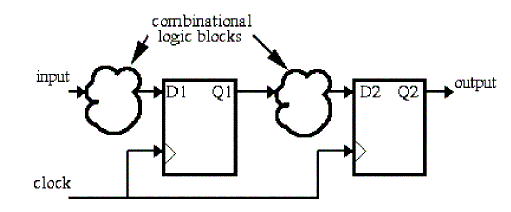Click to view solution

53)Give the circuit to extend the falling edge of the input by 2 clock pulses?The waveforms are shown in the following figure.Click to view solution

51)Design a FSM (Finite State Machine) to detect a sequence 10110?

Click to view solution

41)The question is to design minimal hardware system, which encrypts 8-bit parallel data. A synchronized clock is provided to this system as well. The output encrypted data should be at the same rate as the input data but no necessarily with the same phase?The encryption system is centered around a memory device that perform a LUT (Look-Up Table) conversion. This memory functionality can be achieved by using a PROM, EPROM, FLASH and etc. The device contains an encryption code, which may be burned into the device with an external programmer. In encryption operation, the data_in is an address pointer into a memory cell and the combinatorial logic generates the control signals. This creates a read access from the memory. Then the memory device goes to the appropriate address and outputs the associate data. This data represent the data_in after encryption.

41) What is an LFSR .List a few of its industry applications.?

LFSR is a linear feedback shift register where the input bit is driven by a linear function of the overall shift register value. coming to industrial applications, as far as I know, it is used for encryption and decryption and in BIST(built-in-self-test) based applications..

42)what is false path?how it determine in ckt? what the effect of false path in ckt?

By timing all the paths in the circuit the timing analyzer can determine all the critical paths in the circuit. However, the circuit may have false paths, which are the paths in the circuit which are never exercised during normal circuit operation for any set of inputs.
An example of a false path is shown in figure below. The path going from the input A of the first MUX through the combinational logic out through the B input of the second MUS is a false path. This path can never be activated since if the A input of the first MUX is activated, then Sel line will also select the A input of the second MUX.
STA (Static Timing Analysis) tools are able to identify simple false paths; however they are not able to identify all the false paths and sometimes report false paths as critical paths. Removal of false paths makes circuit testable and its timing performance predictable (sometimes faster)43)Consider two similar processors, one with a clock skew of 100ps and other with a clock skew of 50ps. Which one is likely to have more power? Why?

Clock skew of 50ps is more likely to have clock power. This is because it is likely that low-skew processor has better designed clock tree with more powerful and number of buffers and overheads to make skew better.

44)What are multi-cycle paths?

Multi-cycle paths are paths between registers that take more than one clock cycle to become stable.
For ex. Analyzing the design shown in fig below shows that the output SIN/COS requires 4 clock-cycles after the input ANGLE is latched in. This means that the combinatorial block (the Unrolled Cordic) can take up to 4 clock periods (25MHz) to propagate its result. Place and Route tools are capable of fixing multi-cycle paths problem.45)You have two counters counting upto 16, built from negedge DFF , First circuit is synchronous and second is "ripple" (cascading), Which circuit has a less propagation delay? Why?

The synchronous counter will have lesser delay as the input to each flop is readily available before the clock edge. Whereas the cascade counter will take long time as the output of one flop is used as clock to the other. So the delay will be propagating. For Eg: 16 state counter = 4 bit counter = 4 Flip flops Let 10ns be the delay of each flop The worst case delay of ripple counter = 10 * 4 = 40ns The delay of synchronous counter = 10ns only.(Delay of 1 flop)

46) what is difference between RAM and FIFO?

FIFO does not have address lines
Ram is used for storage purpose where as fifo is used for synchronization purpose i.e. when two peripherals are working in different clock domains then we will go for fifo.

47)The circle can rotate clockwise and back. Use minimum hardware to build a circuit to indicate the direction of rotating.?

2 sensors are required to find out the direction of rotating. They are placed like at the drawing. One of them is connected to the data input of D flip-flop,and a second one - to the clock input. If the circle rotates the way clock sensor sees the light first while D input (second sensor) is zero - the output of the flip-flop equals zero, and if D input sensor "fires" first - the output of the flip-flop becomes high.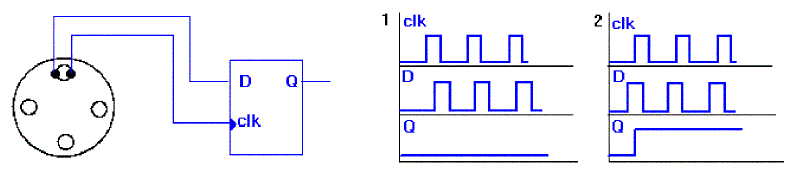49)Implement the following circuits:
(a) 3 input NAND gate using min no of 2 input NAND Gates
(b) 3 input NOR gate using min no of 2 inpur NOR Gates
(c) 3 input XNOR gate using min no of 2 inpur XNOR Gates
Assuming 3 inputs A,B,C?

3 input NAND:
Connect :
a) A and B to the first NAND gate
b) Output of first Nand gate is given to the two inputs of the second NAND gate (this basically realizes the inverter functionality)
c) Output of second NAND gate is given to the input of the third NAND gate, whose other input is C
((A NAND B) NAND (A NAND B)) NAND C Thus, can be implemented using '3' 2-input NAND gates. I guess this is the minimum number of gates that need to be used.
3 input NOR:
Same as above just interchange NAND with NOR ((A NOR B) NOR (A NOR B)) NOR C
3 input XNOR:
Same as above except the inputs for the second XNOR gate, Output of the first XNOR gate is one of the inputs and connect the second input to ground or logical '0'
((A XNOR B) XNOR 0)) XNOR C

50) Is it possible to reduce clock skew to zero? Explain your answer ?

Even though there are clock layout strategies (H-tree) that can in theory reduce clock skew to zero by having the same path length from each flip-flop from the pll, process variations in R and C across the chip will cause clock skew as well as a pure H-Tree scheme is not practical (consumes too much area).

52)Convert D-FF into divide by 2. (not latch)? What is the max clock frequency of the circuit , given the following information?
T_setup= 6nS
T_hold = 2nS
T_propagation = 10nS

Circuit:
Connect Qbar to D and apply the clk at clk of DFF and take the O/P at Q. It gives freq/2.
Max. Freq of operation:
1/ (propagation delay+setup time) = 1/16ns = 62.5 MHz

54) For the Circuit Shown below, What is the Maximum Frequency of Operation?Are there any hold time violations for FF2? If yes, how do you modify the circuit to avoid them?The minumum time period = 3+2+(1+1+1) = 8ns Maximum Frequency = 1/8n= 125MHz.
And there is a hold time violation in the circuit,because of feedback, if you observe, tcq2+AND gate delay is less than thold2,To avoid this we need to use even number of inverters(buffers). Here we need to use 2 inverters each with a delay of 1ns. then the hold time value exactly meets.

55)Design a D-latch using (a) using 2:1 Mux (b) from S-R Latch ?

Click to view solution

56)How to implement a Master Slave flip flop using a 2 to 1 mux?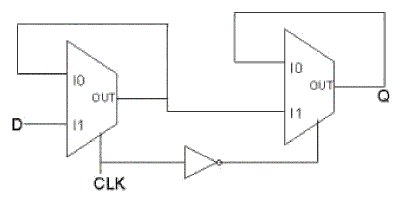57)how many 2 input xor's are needed to inplement 16 input parity generator ?

It is always n-1 Where n is number of inputs.So 16 input parity generator will require 15 two input xor's .

58)Design a circuit for finding the 9's compliment of a BCD number using 4-bit binary adder and some external logic gates?

9's compliment is nothing but subracting the given no from 9.So using a 4 bit binary adder we can just subract the given binary no from 1001(i.e. 9).Here we can use the 2's compliment method addition.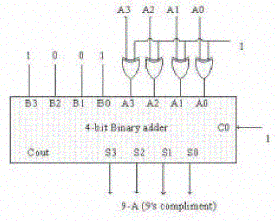59) what is Difference between writeback and write through cache?

A caching method in which modifications to data in the cache aren't copied to the cache source until absolutely necessary. Write-back caching is available on many microprocessors , including all Intel processors since the 80486. With these microprocessors, data modifications to data stored in the L1 cache aren't copied to main memory until absolutely necessary. In contrast, a write-through cache performs all write operations in parallel -- data is written to main memory and the L1 cache simultaneously. Write-back caching yields somewhat better performance than write-through caching because it reduces the number of write operations to main memory. With this performance improvement comes a slight risk that data may be lost if the system crashes.
A write-back cache is also called a copy-back cache.

60)Difference between Synchronous,Asynchronous & Isynchronous communication?

Sending data encoded into your signal requires that the sender and receiver are both using the same enconding/decoding method, and know where to look in the signal to find data. Asynchronous systems do not send separate information to indicate the encoding or clocking information. The receiver must decide the clocking of the signal on it's own. This means that the receiver must decide where to look in the signal stream to find ones and zeroes, and decide for itself where each individual bit stops and starts. This information is not in the data in the signal sent from transmitting unit.

Synchronous systems negotiate the connection at the data-link level before communication begins. Basic synchronous systems will synchronize two clocks before transmission, and reset their numeric counters for errors etc. More advanced systems may negotiate things like error correction and compression.

Time-dependent. it refers to processes where data must be delivered within certain time constraints. For example, Multimedia stream require an isochronous transport mechanism to ensure that data is delivered as fast as it is displayed and to ensure that the audio is synchronized with the video.

61) What are different ways Multiply & Divide?

Click to view solution

Verilog FAQ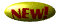Synthesis FAQ

Digital FAQ

Timing FAQ

ASIC FAQCmos FAQ

Misc FAQ

Home